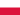Marginal Revenue Below Average Total CostAll Modules View all topics Close topics
Module 1: Understanding Fixed, Variable and Marginal Costs in Economics

# Marginal Revenue Below Average Total Cost

• Study Reminders

We'll email you at these times to remind you to study

You can set up to 7 reminders per week

#### You're all set

We'll email you at these times to remind you to study

Monday

Tuesday

Wednesday

Thursday

Friday

Saturday

Sunday

• Study Notes
• Discuss This TopicKaram A. 0 0 here i want to explain the reason ,,Why is marginal revenue below average revenue for a monopolist? A mathematical connection between average revenue and marginal revenue stating that the change in the average revenue depends on a comparison between average revenue and marginal revenue. For perfect competition, with no market control, marginal revenue is equal to average revenue, and average revenue does not change. For monopoly and other firms with market control, marginal revenue is less than average revenue, and average revenue falls. The relation between average revenue and marginal revenue',500,400)">marginal revenue is one of several that reflect the general relation between a marginal and the corresponding average. The general relation is this: If the marginal is less than the average, then the average declines. If the marginal is greater than the average, then the average rises. If the marginal is equal to the average, then the average does not change. This general relation surfaces throughout the study of economics. It also applies to average and marginal product, average and marginal cost, average and marginal factor cost, average and marginal propensity to consume, and well, any other average and marginal encountered in economics.
• Text Version